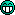^ 回到顶部
• 人生没有定律，每个人都有自己的节奏
• 本站wordpress建站教程均通过实践后发布，希望对你有帮助，如果有代码出错，请联系站长解决
• 希望你的坚持，都是因为热爱，而不是因为不甘心
• 8年wordpress建站经验，5星服务品质
• 那些不愿意让你吃亏的人，才是真正值得你深交的人，也是值得你付出时间的人
• 阿里云ECS服务器

# 零起点php入门第15课-php数组（4）

1、数组排序

```<?php
\$arr=array(35,43,56,2,76,23,47,55,71);		//定义一个数组
echo '数组排序之前的信息：<br />';
print_r(\$arr);		//输出排序前的数组信息
for(\$i=0;\$i<count(\$arr);\$i++){		//对数组进行排序
for(\$j=0;\$j<count(\$arr)-1;\$j++){
if(\$arr[\$j]>\$arr[\$j+1]){		//判断前后元素的大小
\$b=\$arr[\$j];		//交换元素的值
\$arr[\$j]=\$arr[\$j+1];
\$arr[\$j+1]=\$b;
}
}
}
echo '<br />数组排序之后的信息：<br />';
print_r(\$arr);		//输出排序后的数组信息
?>```

Array (  => 35  => 43  => 56  => 2  => 76  => 23  => 47  => 55  => 71 )

Array (  => 2  => 23  => 35  => 43  => 47  => 55  => 56  => 71  => 76 )

```<?php
function mysort(\$arr){		//将排序的代码封装为函数
echo '<br />数组排序之前的信息：<br />';
print_r(\$arr);		//输出排序前的数组信息
for(\$i=0;\$i<count(\$arr);\$i++){		//对数组进行排序
for(\$j=0;\$j<count(\$arr)-1;\$j++){
if(\$arr[\$j]>\$arr[\$j+1]){
\$b=\$arr[\$j];
\$arr[\$j]=\$arr[\$j+1];
\$arr[\$j+1]=\$b;
}
}
}
echo '<br />数组排序之后的信息：<br />';
print_r(\$arr);		//输出排序前的数组信息
}
\$arr1=array(654,853,123,147,259,377);		//定义数组
\$arr2=array(23,45,1,12,22,90,145,75);
\$arr3=array(123,23,741,638,254,36,11);
mysort(\$arr1);		//使用自定义函数排序
mysort(\$arr2);		//使用自定义函数排序
mysort(\$arr3);		//使用自定义函数排序
?>```

```数组排序之前的信息：
Array (  => 654  => 853  => 123  => 147  => 259  => 377 )

Array (  => 123  => 147  => 259  => 377  => 654  => 853 )

Array (  => 23  => 45  => 1  => 12  => 22  => 90  => 145  => 75 )

Array (  => 1  => 12  => 22  => 23  => 45  => 75  => 90  => 145 )

Array (  => 123  => 23  => 741  => 638  => 254  => 36  => 11 )

Array (  => 11  => 23  => 36  => 123  => 254  => 638  => 741 )```

bool sort ( array &\$array [, int \$sort_flags ] )  //用于对数组正向（从小到大）排序

bool rsort ( array &\$array [, int \$sort_flags ] )  //用于对数组逆向（从大到小）排序

SORT_REGULAR：正常比较元素（默认方式）；

SORT_NUMERIC：元素被作为数字来比较；

SORT_STRING：元素被作为字符串来比较。

```<?php
\$arr=array(2,54,167,'a','A','12');		//定义一个数组
echo '数组排序之前的信息：<br />';
print_r(\$arr);		//输出数组的信息
echo '<br />数组普通排序之后的信息：<br />';
sort(\$arr);			//对数组进行排序
print_r(\$arr);
echo '<br />数组作为数字排序之后的信息：<br />';
sort(\$arr,SORT_NUMERIC);		//将数组的元素转换为数字进行排序
print_r(\$arr);
echo '<br />数组作为字符排序之后的信息：<br />';
sort(\$arr,SORT_STRING);		//将数组的元素转换为字符进行排序
print_r(\$arr);
?>
```

Array (  => 2  => 54  => 167  => a  => A  => 12 )

Array (  => A  => a  => 2  => 12  => 54  => 167 )

Array (  => a  => A  => 2  => 12  => 54  => 167 )

Array (  => 12  => 167  => 2  => 54  => A  => a )

sort 和 rsort 函数的使用和理解都比较简单，陌小雨就不再做详细讲解了。

bool shuffle ( array &\$array )

```<?php
\$arr=array(3,23,'A','f','123','hello');		//定义一个数组
echo '排序之前的数组信息：<br />';
print_r(\$arr);
shuffle(\$arr);		//对数组进行随机排序
echo '<br />排序之后的数组信息：<br />';
print_r(\$arr);		//输出数组信息
?>
```

Array (  => 3  => 23  => A  => f  => 123  => hello )

Array (  => f  => A  => hello  => 23  => 123  => 3 )

2、过滤数组元素

```<?php
function myfunc(&\$arr){		//自定义一个过滤函数
\$j=count(\$arr);
for(\$i=0;\$i<\$j;\$i++){
if(\$arr[\$i]%2==0)
unset(\$arr[\$i]);
}
}
\$arr=array(23,14,37,263,244,379,100,153,150);		//定义一个数组
echo '数组进行过滤之前的信息：<br />';
print_r(\$arr);
myfunc(\$arr);		//调用自定义函数
echo '<br />数组进行过滤之后的信息：<br />';
print_r(\$arr);
?>```

Array (  => 23  => 14  => 37  => 263  => 244  => 379  => 100  => 153  => 150 )

Array (  => 23  => 37  => 263  => 379  => 153 )

```<?php
function myfunc(\$arr){		//定义过滤函数
\$j=count(\$arr);
for(\$i=0;\$i<\$j;\$i++){
if(\$arr[\$i]>=0&&\$arr[\$i]<=100)
\$n_arr[]=\$arr[\$i];
}
return \$n_arr;
}
\$arr=array(2,34,5,175,168,94,942,105);
echo '原来的数组信息：<br />';
print_r(\$arr);
\$newarr=myfunc(\$arr);		//调用函数并使用变量接收函数的返回值
echo '<br />过滤出来的新数组信息：<br />';
print_r(\$newarr);		//输出新数组的信息
?>```

Array (  => 2  => 34  => 5  => 175  => 168  => 94  => 942  => 105 )

Array (  => 2  => 34  => 5  => 94 )

array array_filter ( array \$input [, callback \$callback ] )

```<?php
function odd(\$x){		//定义过滤偶数的函数
if(\$x%2==1)
return TRUE;
}
function even(\$x){		//定义过滤奇数的函数
if(\$x%2==0)
return TRUE;
}
\$arr=array(1,2,3,123,35,47,58,103,116);			//定义一个数组
echo '过滤前的数组信息：<br />';
print_r(\$arr);
echo '<br />过滤掉偶数后的数组信息：<br />';
print_r(array_filter(\$arr,'odd'));			//调用函数对数组进行过滤
echo '<br />过滤掉奇数后的数组信息：<br />';
print_r(array_filter(\$arr,'even'));
?>
```

Array (  => 1  => 2  => 3  => 123  => 35  => 47  => 58  => 103  => 116 )

Array (  => 1  => 3  => 123  => 35  => 47  => 103 )

Array (  => 2  => 58  => 116 )

```<?php
function not_int(\$x){		//定义过滤非整数的函数
if(is_int(\$x))
return TRUE;
}
\$arr=array(1,2,'a',3,4,'c','d');		//定义数组
echo '过滤前的数组信息：<br />';
print_r(\$arr);		//输出过滤前的数组信息
\$res=array_filter(\$arr,'not_int');		//调用函数过滤数组
echo '<br />过滤后的数组信息：<br />';
print_r(\$res);		//输出过滤后的数组信息
?>
```

Array (  => 1  => 2  => a  => 3  => 4  => c  => d )

Array (  => 1  => 2  => 3  => 4 )### 如有疑问，请前往问答中心反馈！

1.憧憬Licoy
小雨写的很全，不错的• 陌小雨网站管理员
@憧憬Licoy 如果分享的文章对你有用，诚邀你去百度口碑点个赞哦，也是对小雨的肯定和鼓励！